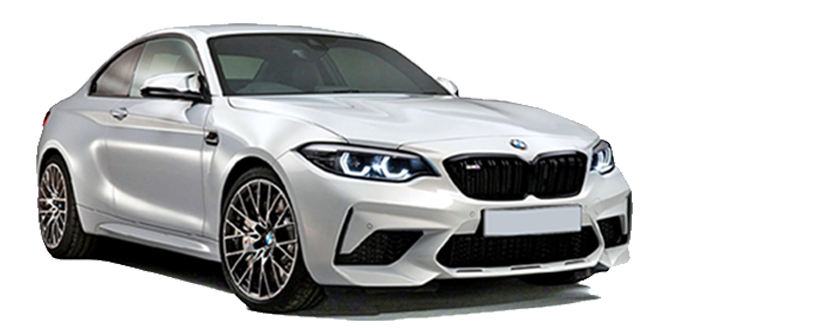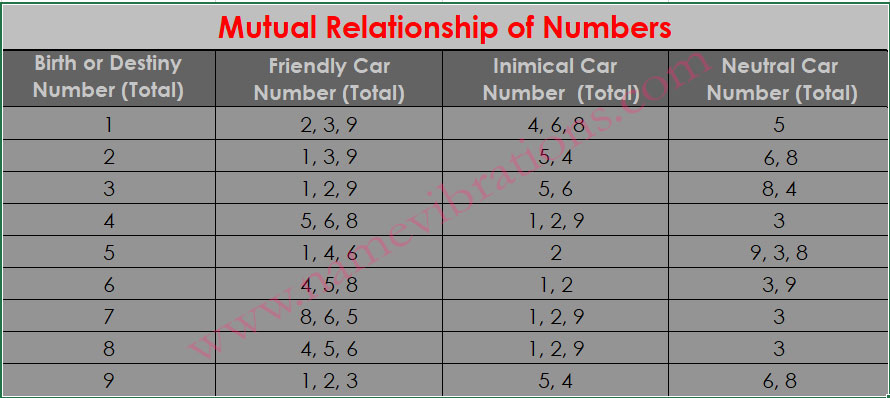+1 (705) 906-9693 contact@namevibrations.com
Select Page

# Lucky License Plate Calculator For Vehicle

What is a Lucky License Plate for Vehicle?

Your License Plate for Vehicles should be friendly (in harmony) to either your Lucky Number, Birth Number,  Destiny Number, or all of them.

Put your date of birth and prospective license plate number in our Lucky License Plate Calculator For Vehicle astrological tool to match your car number with your Birth Number or Destiny Number. Our unique tool provides calculations for both Chaldean & Pythagorean values of the license plate. So, that you can easily evaluate if your license plate is favourable on one system or both.

- -

### Date Of Birth Number - //

BIRTH NUMBER:
DESTINY OR LIFE PATH NUMBER:

CHALDEAN :
PYTHAGOREAN :

## How Does Our License Plate Calculator work?

1. The online tool calculates your birth number and destiny number.
2. Converts the letters of the License Plate into numbers.
3. Adds the numerical value of the letters and digits in the license plate of the vehicle.
4. Finally, brings that total to a single digit.

NOTE: That single-digit value of the license plate must match with your birth (psychic) number or destiny (life path) number.

To find out if your license plate is compatible with your DOB or not, please check the Mutual Relationship of Numbers (Friendship or Enmity Chart) below, and to know how to calculate it manually, just scroll down and refer to the Chaldean & Pythagorean numerical value of the letters.Go for a Licence Plate that vibrates at higher frequencies and proves Lucky for you based on your Date of Birth

## How To Read The Results?

There can be four instances in matching the license plate to the birth number or destiny number:

1. Both Chaldean & Pythagorean license plate numbers are friendly to the birth number and/or destiny number. (amazing compatibility)
2. Both the Chaldean and Pythagorean values of the license plate are not friendly to the birth number and/or destiny number. (worst compatibility)
3. Only the Chaldean value of the license plate is friendly or neutral, but the Pythagorean value of the license plate is neither friendly nor neutral to the birth number and/or destiny number. (good compatibility)
4. Chaldean does not match, but only Pythagorean matches the birth number and/or destiny number. (low compatibility)

Check License Plates with Strong Vibrations here

If you already own a vehicle with a license plate that is neutral to your DOB (birth number & destiny number), then it is still acceptable. However, if the license plate number is not friendly to your DOB, then you must change the license plate.### Numerical Value of Alphabets (Chaldean & Pythagorean)

Chaldean :  AIJQY = 1, BKR = 2, CGLS = 3, DMT = 4, EHNX = 5, UVW = 6, OZ = 7, FP = 8

Pythagorean : AIS = 1, BKT = 2, CGU = 3, DMV = 4, EHW = 5, FOX = 6, GPY = 7, HQZ = 8, IR = 9

### How To Calculate Manually (Chaldean)

Birth Number 1

Here is a vehicle number DL 03 XB 1234. So according to the above steps.

1. DL = 43 and XB = 52
2. (0+3) + (1+2+3+4)
3. (4+3) + (5+2) + (0+3) + (1+2+3+4)
4. 7 + 7 + 3 + 10 = 28 = 10 = 9

Basic rule is take the total of the numbers (1+2+3+4)  = 10 = 1

My personal experience is most of the time if total of the number is friendly (1+2+3+4)  = 10 = 1

Chances are more that entire number comes out friendly (DL XB 03 1234) = (4+3) + (5+2) + (0+3) + (1+2+3+4) = 9

Here Total of numbers = 1 & Total of entire number = 9 (Both Friendly to Birth Number 1)

In case it is not, even if you have to pay some fees or wait for some time, get a number that is friendly (in harmony) to your Birth Number.